# Inflation

MPQ theory wednesday seminar by Lucas Clemente

2014-04-09

# I'm not an expert!

### Stuff will be wrong.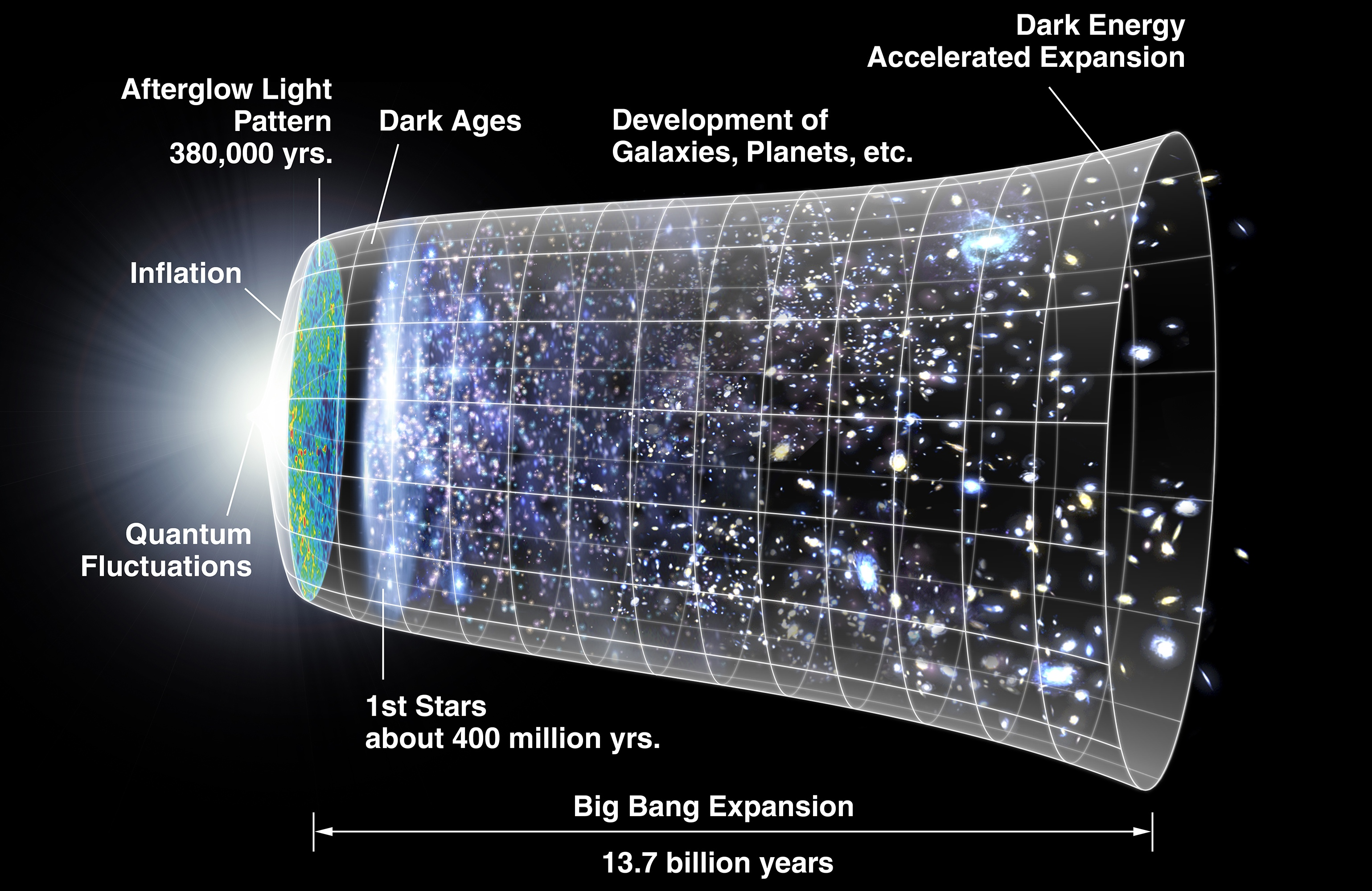Image by NASA WMAP / Public Domain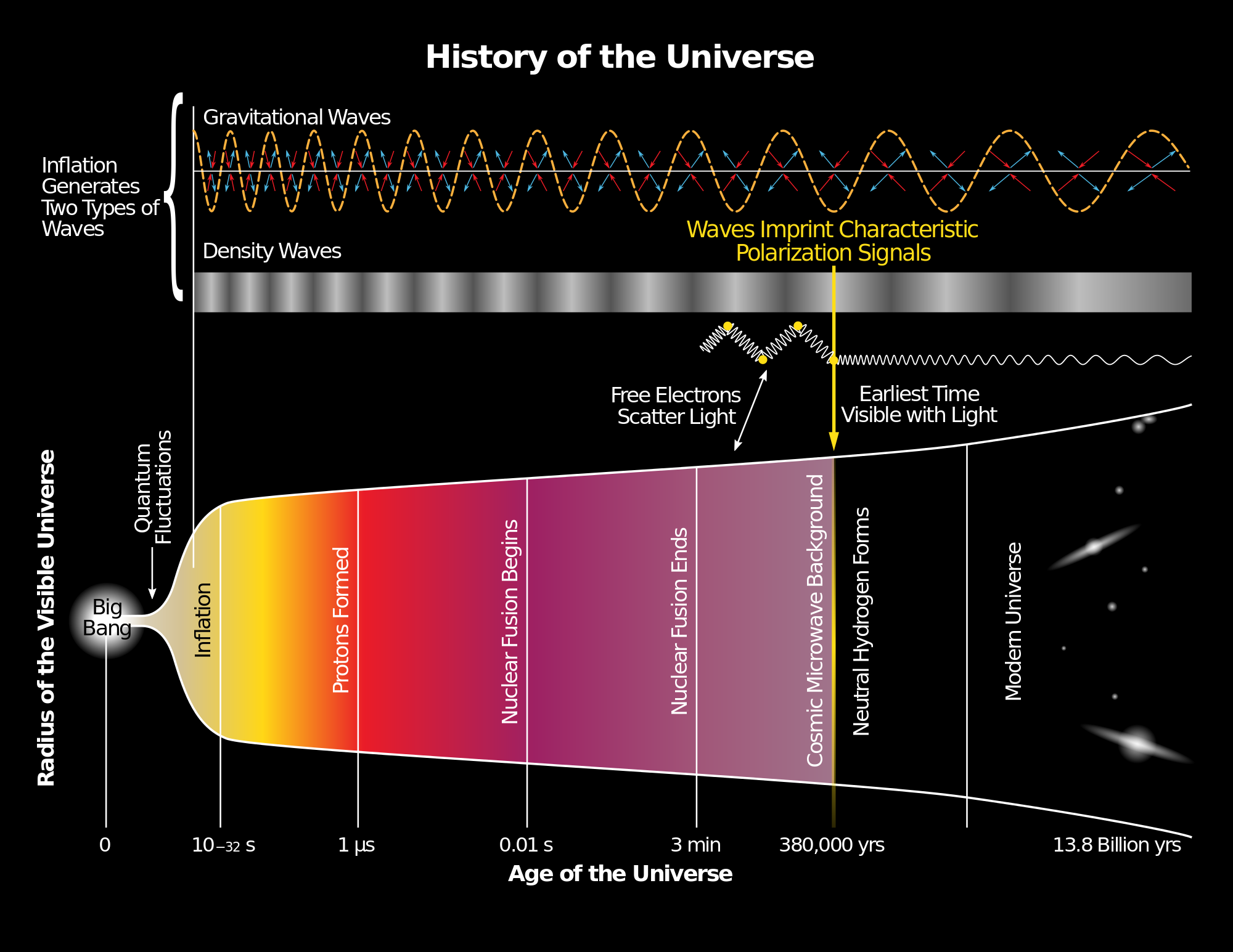Image by Yinweichen / CC BY SA

## Observation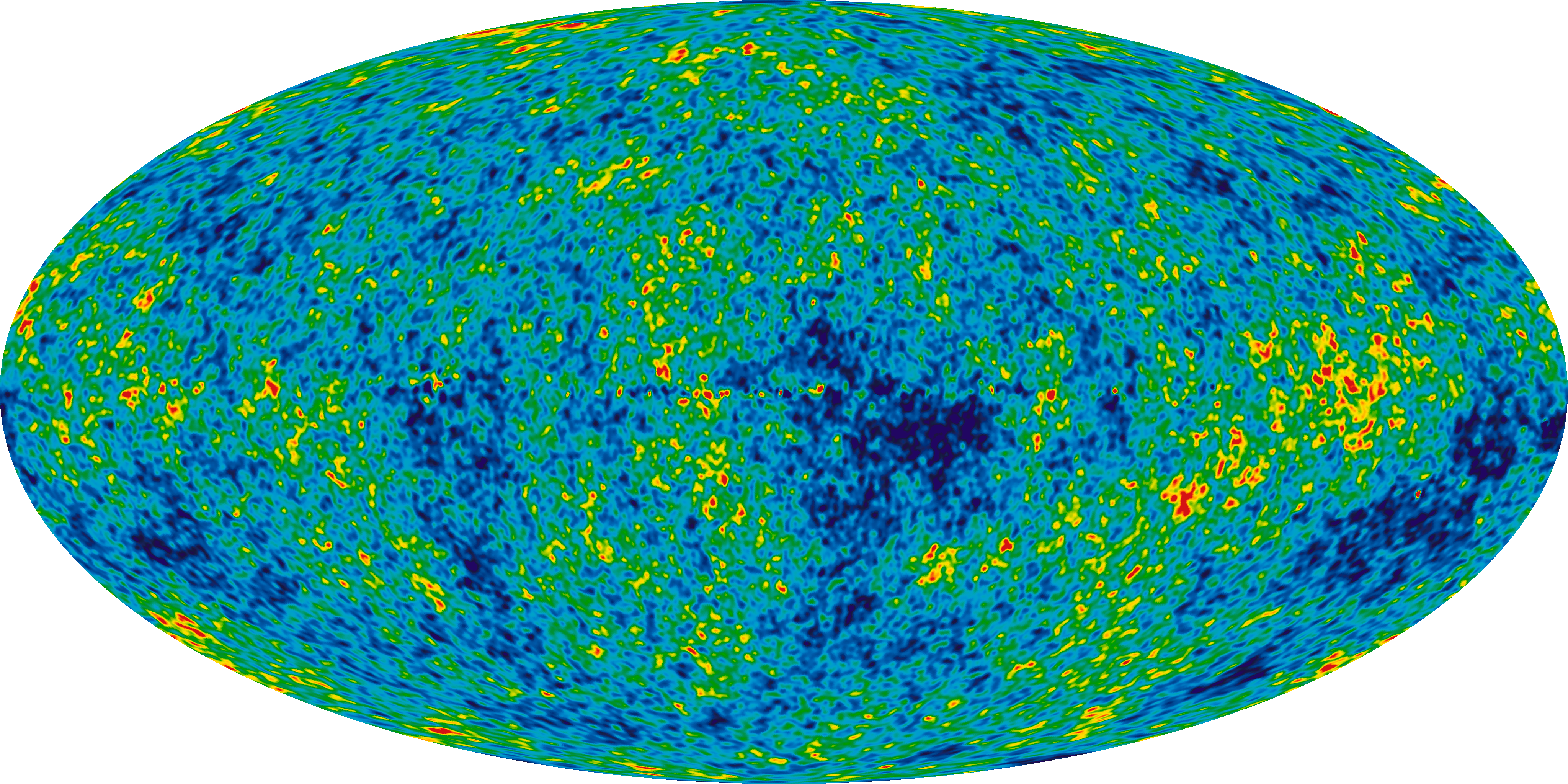Cosmic Microwave Background (CMB)

Image by NASA / Public Domain

## Comoving coordinates

comoving observers see an isotropic universe / CMB

conformal time: time of comoving observers

comoving distance: constant between two comoving objects

## FLRW Metric

$ds^2 = - dt^2 + a^2(t) (dx^2 + dy^2 + dz^2)$ scale factor $a(t)$, $a(\text{today}) \equiv 1$

$ds^2 = a^2(t) (- d\tau^2 + (dx^2 + dy^2 + dz^2))$

## Hubble

Hubble's law: $v = H_0 D$

Hubble parameter $H = \frac{\dot a}{a}$

Hubble radius $(H)^{-1}$

comoving Hubble radius $(a H)^{-1}$

## Three lengths

Radius of the observable universe $\tau$: 46 Gly

Age of the universe: 13.8 Gy

Hubble radius (today) $H_0^{-1}$: 14 Gly larger due to dark energy

## Particle horizon

$\tau = \int d\tau = \int_0^t \frac{dt'}{a(t')} = \int_0^a (a' H)^{-1} d \ln a'$

maximum distance light can have travelled in time $t$

## separation by ...

... comoving Hubble radius $(a H)^{-1}$: can't talk now

... comoving horizon $\tau$: could never have talked

## different types of universe

decelerating universe: $(a H)^{-1}$ growing

accelerating universe: $(a H)^{-1}$ decreasing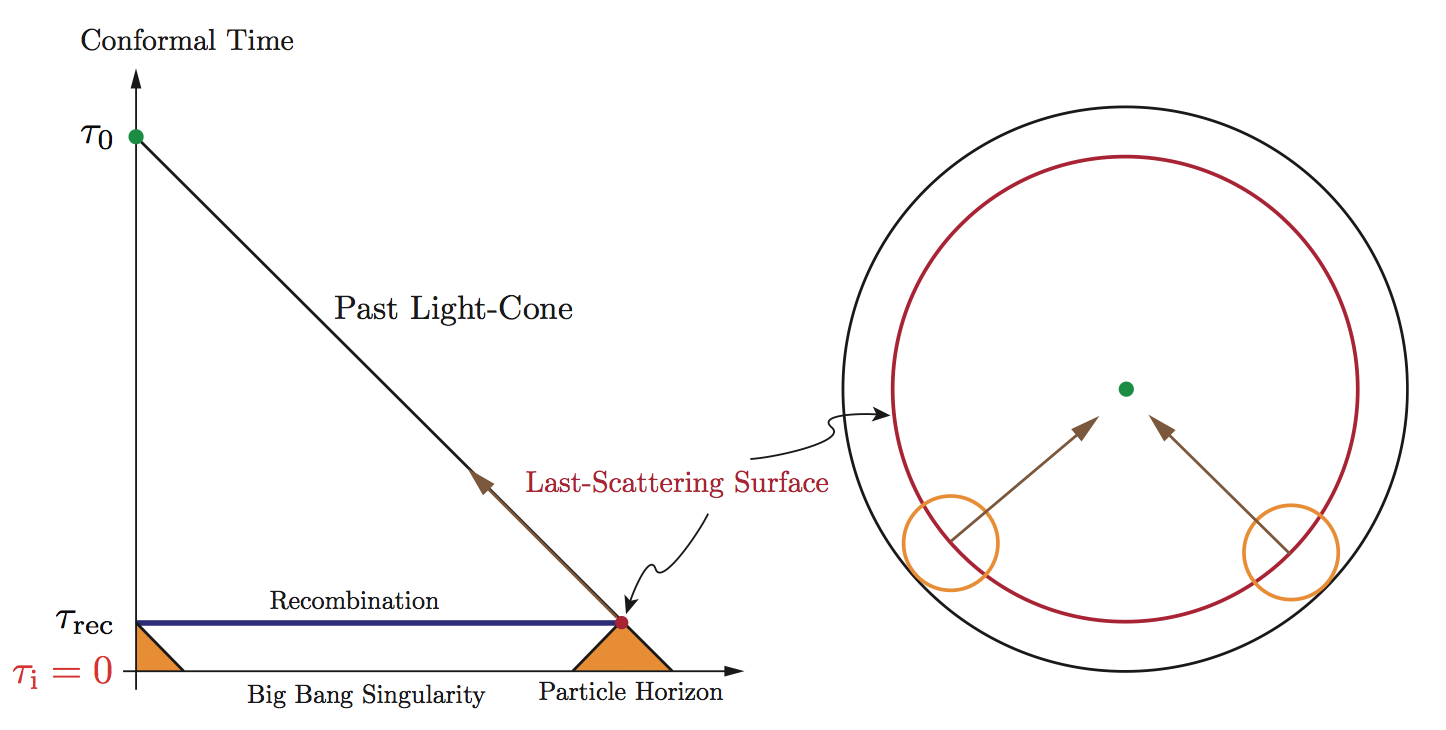Image by Daniel Baumann / arXiv:0907.5424

# Horizon Problem

### If $(a H)^{-1}$ grows in time...

... stuff entering the comoving Hubble volume today
cannot have been in contact before!

... but the CMB is in thermal equilibrium!

## Curvature of space

Friedmann Equation: $H^2 = \frac 1 3 \rho(a) - \frac{k}{a^2}$ where $k$: curvature (flat is $k=0$)

$1 - \frac{\rho(a)}{\rho_c(a)} = 1 - \Omega(a) = - \frac{k}{(aH)^2}$

Critical density: ~5 hydrogen per m3 Ordinary matter: ~0.2 per m3, rest is "dark"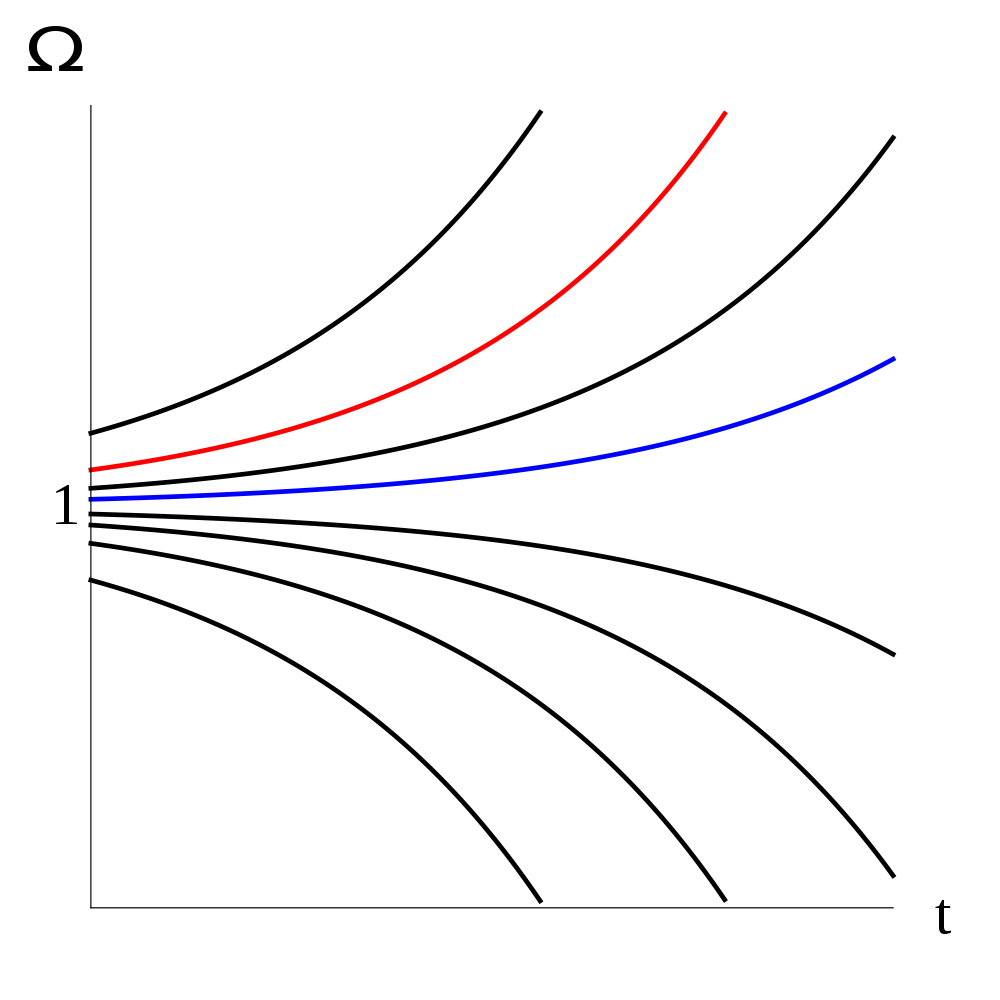Image by Olaf Davis / Public Domain

Alan Guth, 1980

# Inflation

comoving Hubble radius decreases $d_t (aH)^{-1} < 0$ Hubble radius $H^{-1}$ is constant

scale factor increases $\ddot a > 0$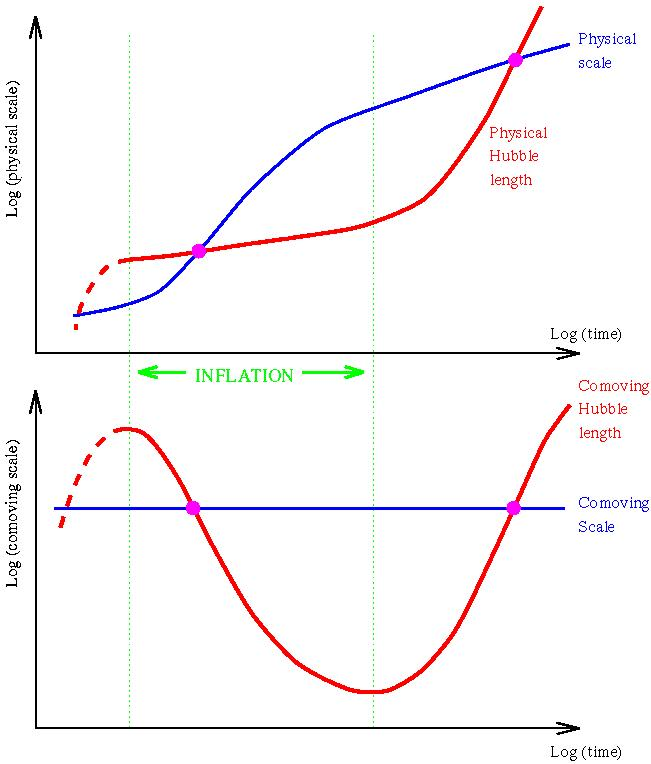Image by Andrew Liddle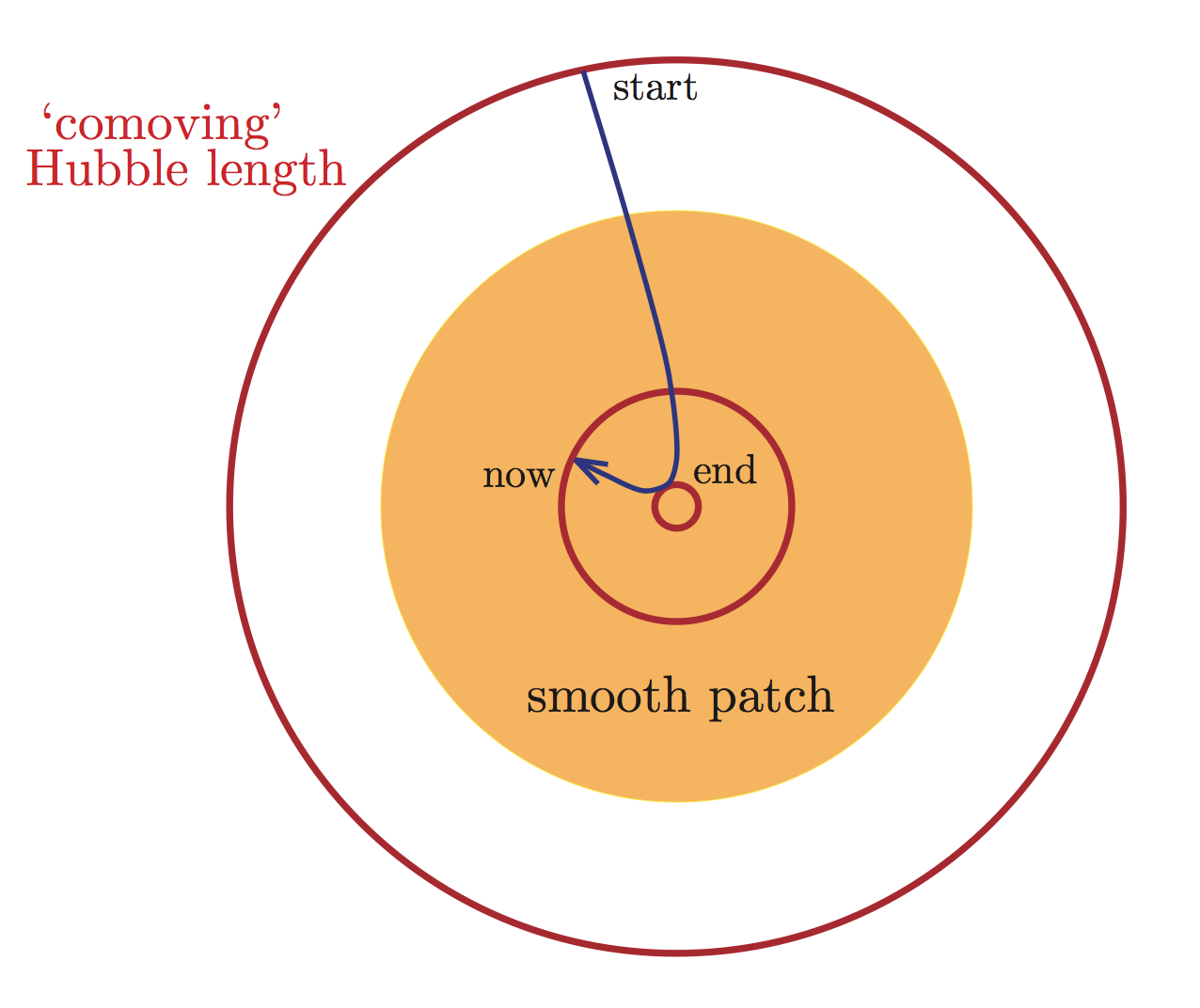Image by Daniel Baumann / arXiv:0907.5424

# solved

$1 - \Omega(a) = - \frac{k}{(aH)^2}$

# Slow-roll inflation

Andrei Linde, 1982

### Inflaton field $V(\phi)$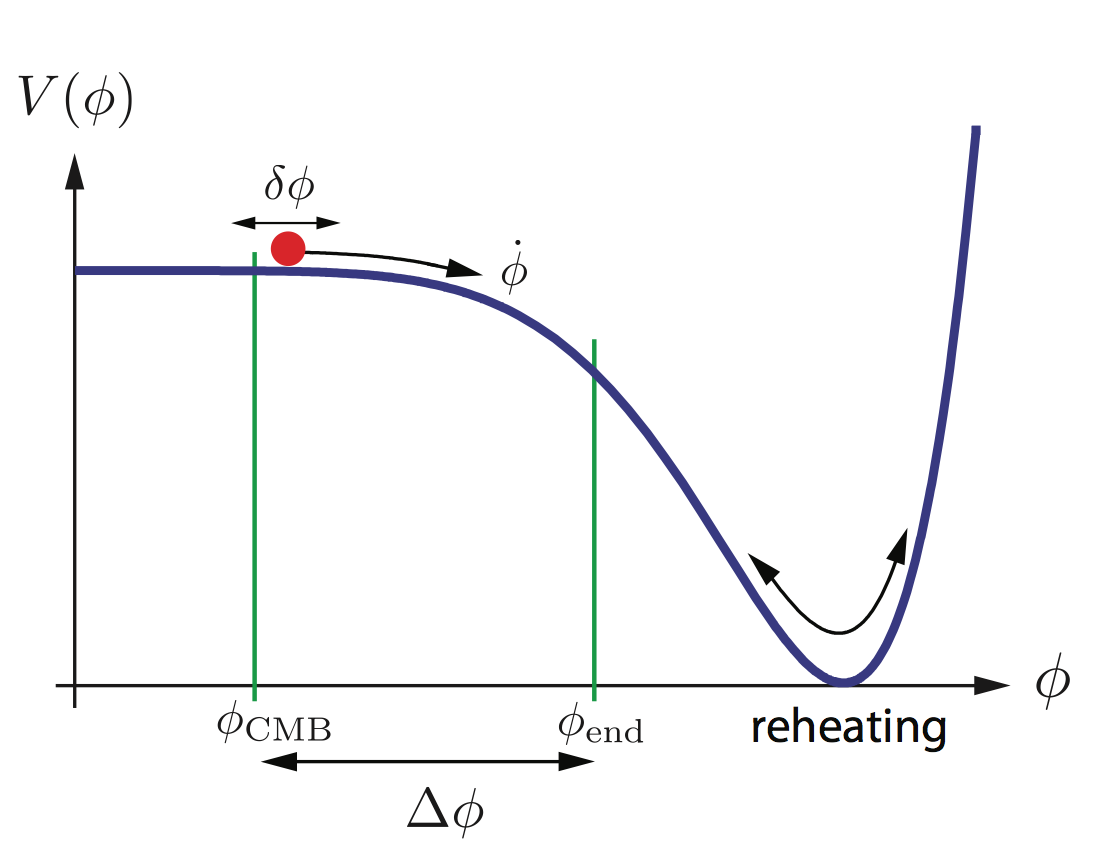Image by Daniel Baumann / arXiv:0907.5424

## Slow-rolling inflation

Friedmann equation $H^2 = \left(\frac{\dot a}{a}\right)^2 = \frac{V(\phi)}{3 m_P}$

If $\phi$ is slow-rolling, $V(\phi) \sim const$,

then $a(t) \sim e^{H t}$ inflates.

## Quantum fluctuations

inflaton field fluctuates with wavelength $\lambda \sim e^{Ht}$

freezes in once $\lambda \sim (a H)^{-1}$

$\delta \phi \sim H$

## Scalar and tensor fluctuations

energy density: $\delta_S^2 = \frac{1}{m_P} \frac{V^3}{V'^2}$

gravitational waves: $\delta_T^2 = \frac{V}{m_P^4}$

## E- and B-modes

fluctuations imprint polarization onto CMB

scalar fluctuations ($\delta_S$): curl-free (E-modes)

tensor fluctuations ($\delta_T$): curl (B-modes)

# BICEP2

$r = \frac{\delta_T^2}{\delta_S^2} = 0.2 \substack{+0.07\\-0.05}$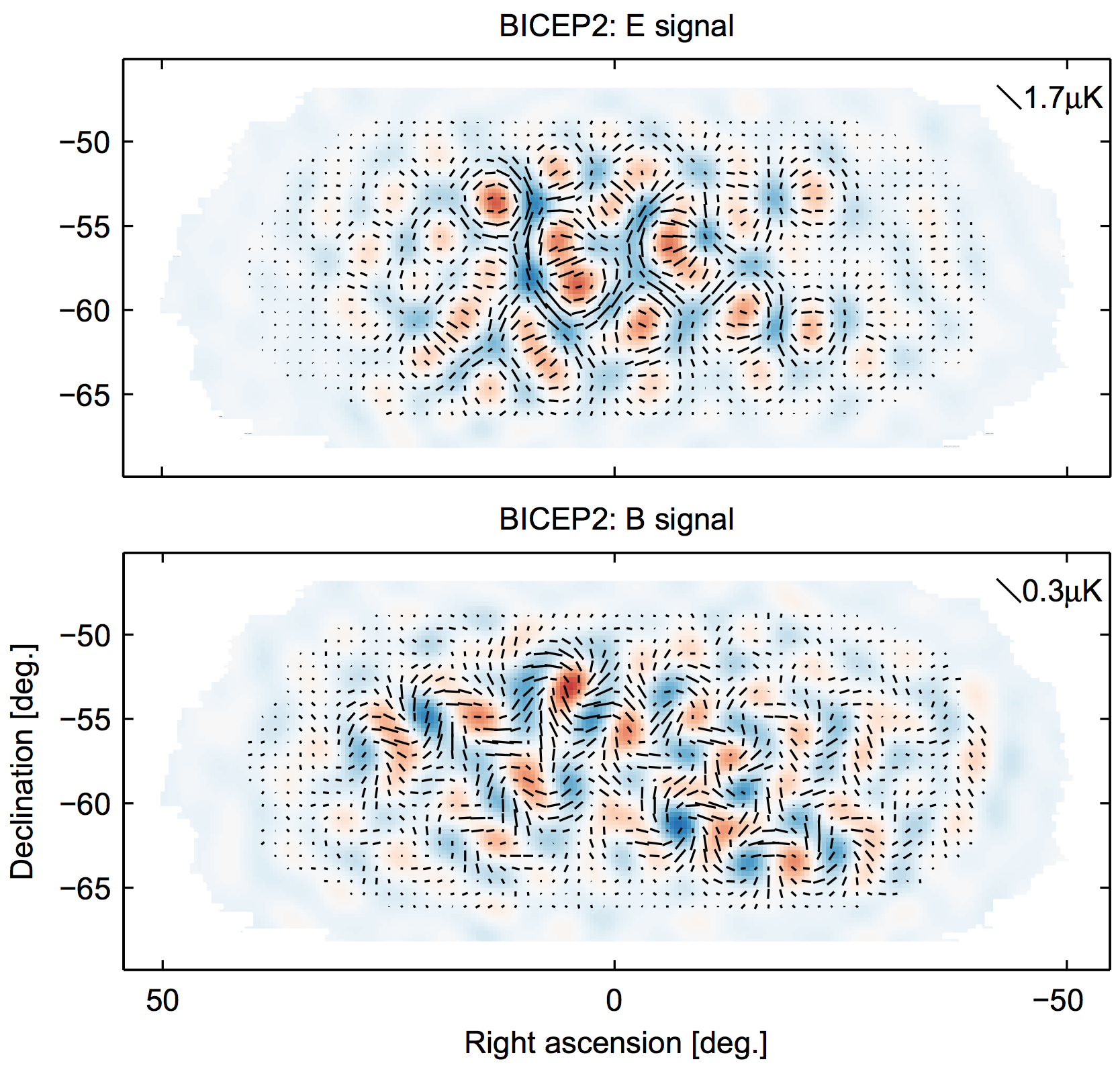Image by BICEP2 / arXiv:1403.3985

## planck scale

$r = 0.2$ allows determining $V \sim 10^{16} \text{GeV}$

reminder that $m_P \sim 10^{19} \text{GeV}$

inflation is close to the Planck-scale
where we could expect GUT effects

## Observation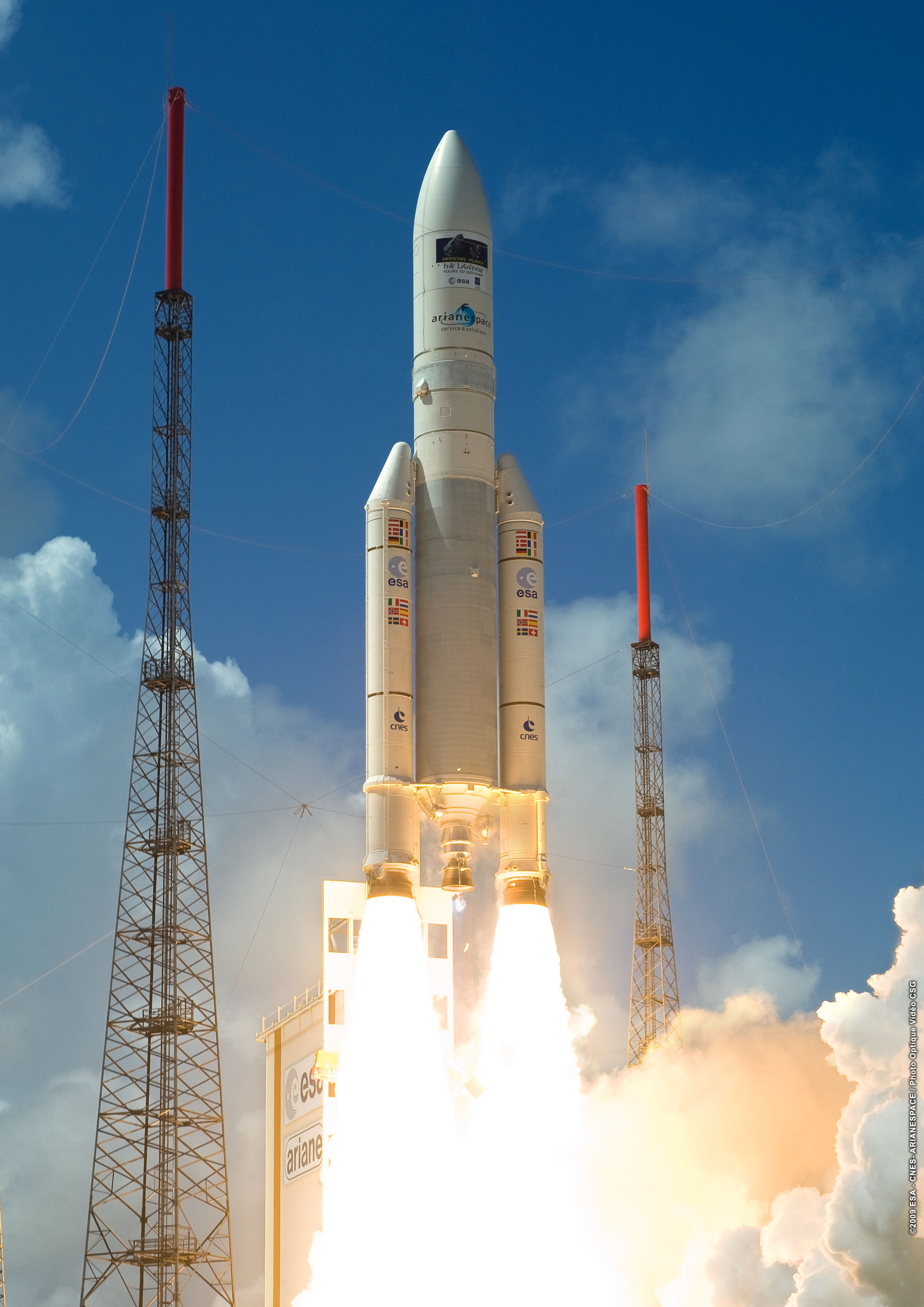Photo by ESA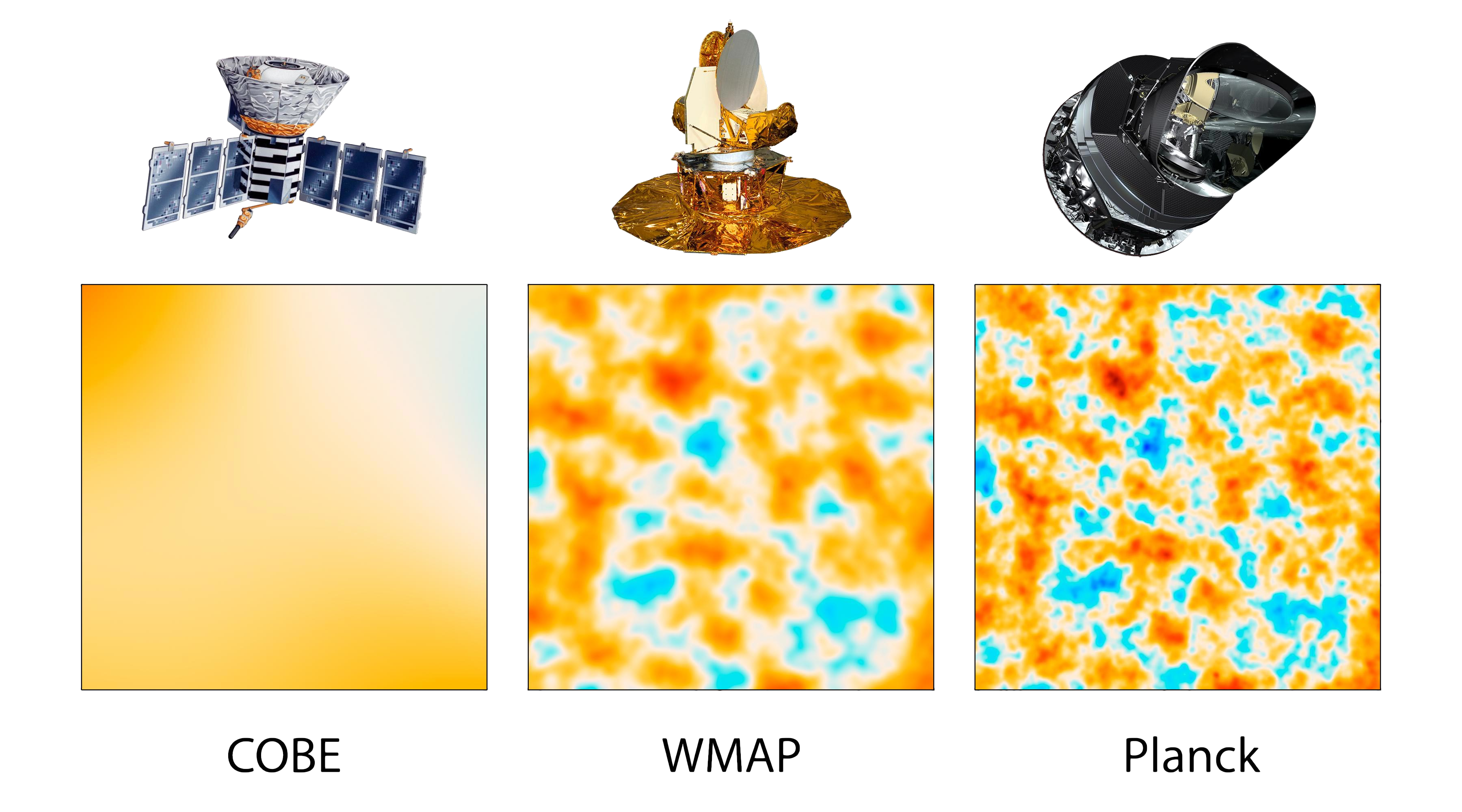Image by NASA / Public Domain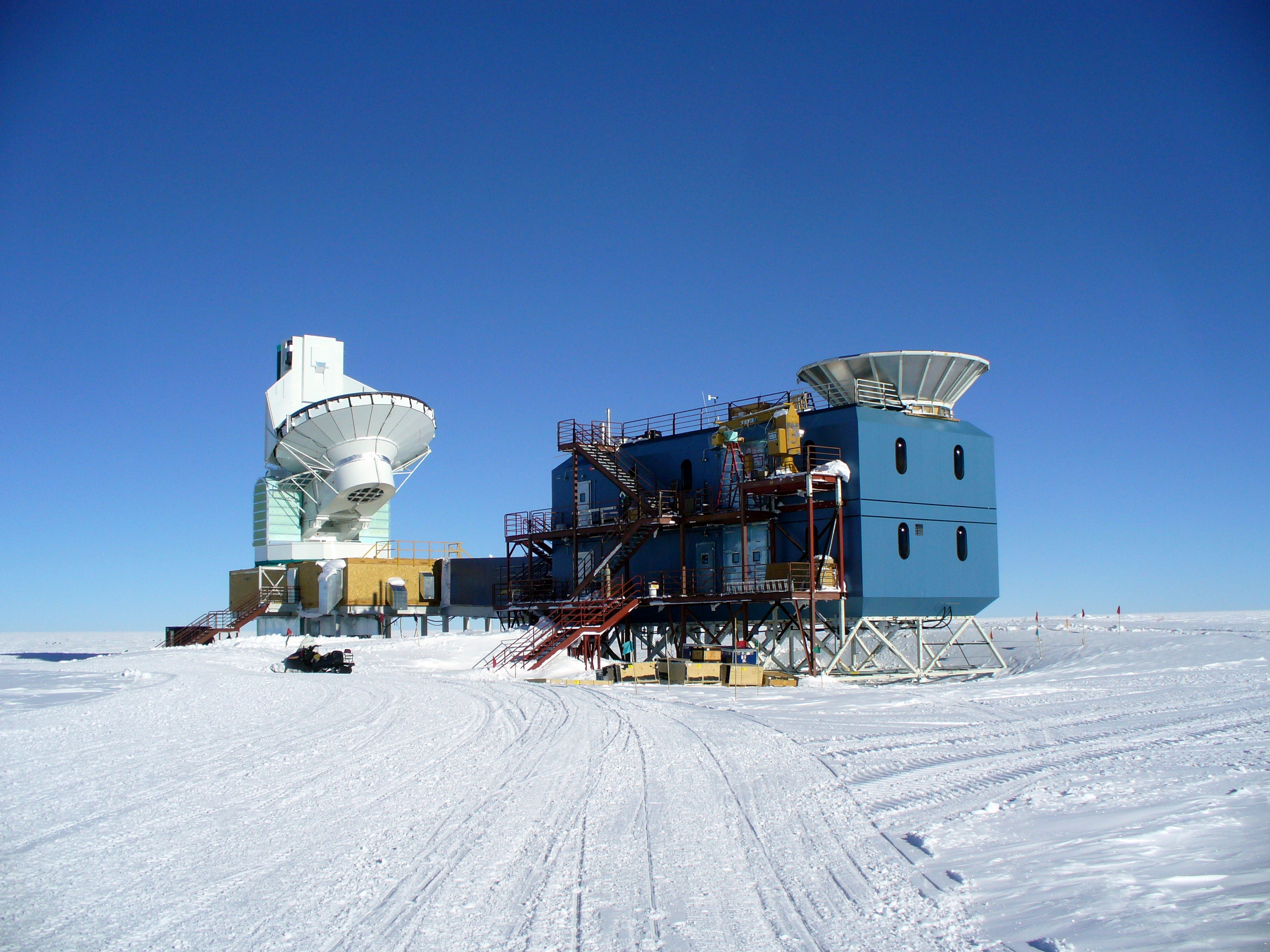Photo by Amble / CC BY SA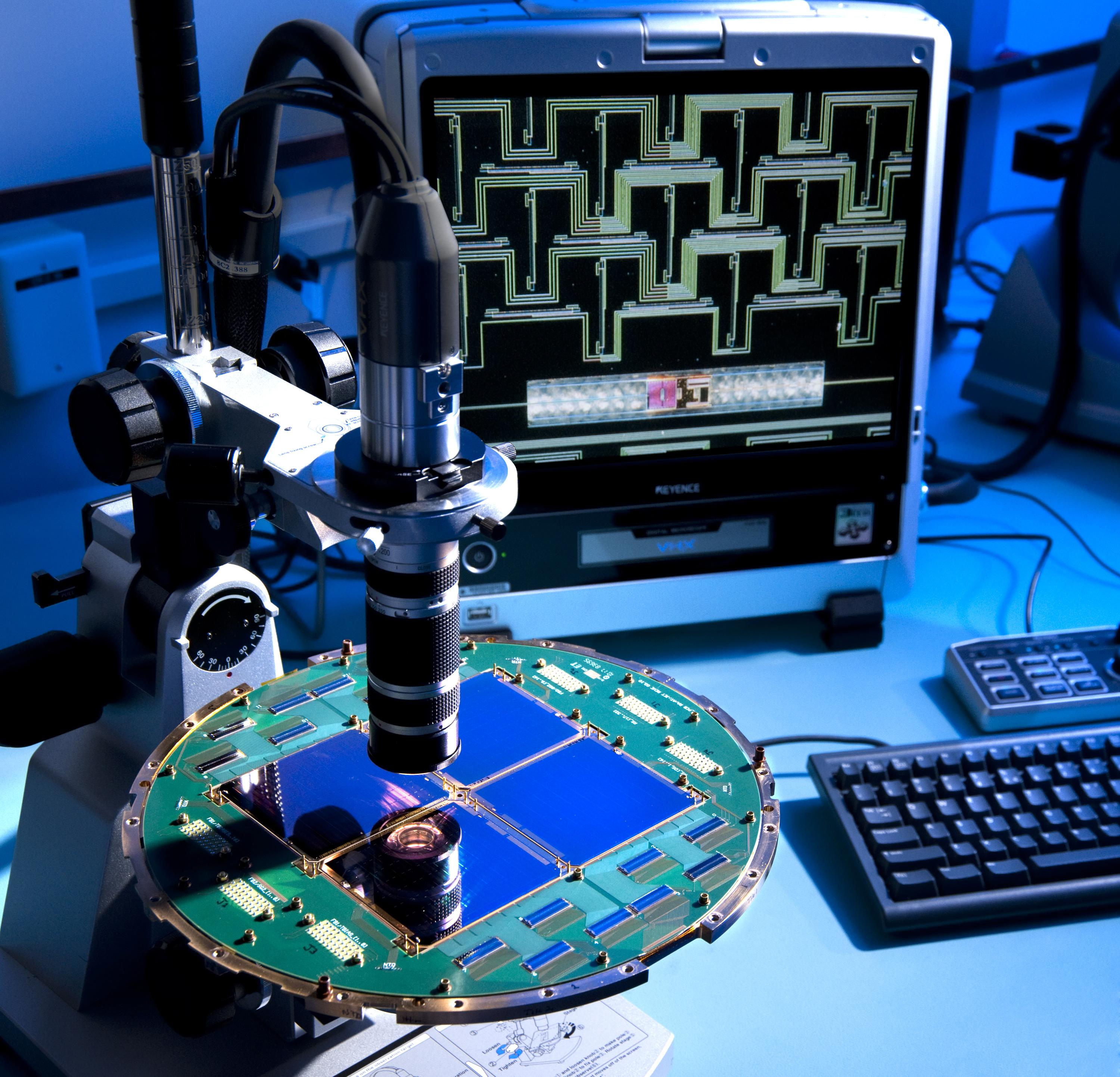Photo by NASA / Public Domain

## TRICEP (Science, 04/14)

Tachyonic Retrospective Inferences of Cosmologically Extrapolated Preconditions

"... used a telescope outfitted with a revolutionary \$11 million detector knitted out of individual superconducting carbon nanotubes, paid for in part by Google and the Roman Catholic Church."

predicted by Einstein in a dream on April 1st 1938!

# THE END

## questions?

once again, clemente.io/inflation